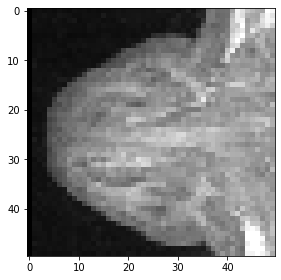# Slicing and cropping#

When working with larger image data, it often makes sense to crop out regions and focus on them for further analysis. For cropping images, we use the same “:”-syntax, we used when indexing in lists and exploring multi-dimensional image data.

import numpy as np


image = imread("../../data/Haase_MRT_tfl3d1.tif")

image.shape

(192, 256, 256)


## Slicing#

For visualizing 3D images using scikit-image’s imshow, we need to select a slice to visualize. For example, a Z-slice:

slice_image = image

imshow(slice_image)

<matplotlib.image.AxesImage at 0x2b54f73d340>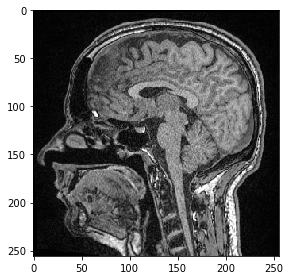We can also select a plane where all pixels have the same Y-position. We just need to specify, that we would like to keep all pixels in Z using the : syntax.

slice_image = image[:, 100]

imshow(slice_image)

<matplotlib.image.AxesImage at 0x2b54f836af0>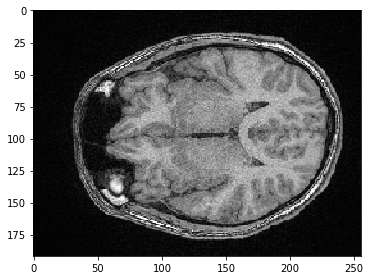## Cropping#

We can also select a sub-stack using indexing in the square brackets.

sub_stack = image[50:150]

sub_stack.shape

(100, 256, 256)


We can also select a sub-region in X. If we want to keep all pixels along Z and Y (the first two dimensions), we just specify : to keep all.

sub_region_x = image[:, :, 100:200]

imshow(sub_region_x)

<matplotlib.image.AxesImage at 0x2b54f8ae850>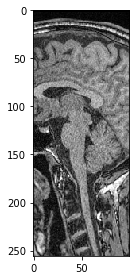For selectinng all pixels in one direction above a given value, we just need to specify the start before :.

sub_region_y = image[:, 100:]

imshow(sub_region_y)

<matplotlib.image.AxesImage at 0x2b54f90ae20>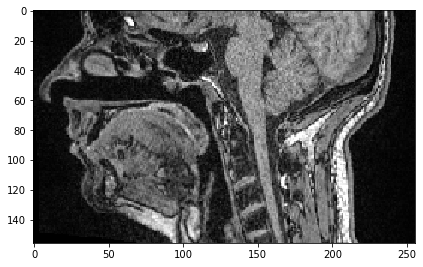Similarly, we can select all pixels up to a given position.

sub_region_x2 = image[:, :, :50]

imshow(sub_region_x2)

<matplotlib.image.AxesImage at 0x2b550943d30>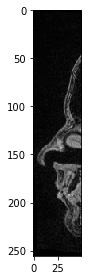Last but not least, this is how a cropped cube is specified.

cropped_cube = image[80:130, 120:170, :50]

cropped_cube.shape

(50, 50, 50)

imshow(cropped_cube)

<matplotlib.image.AxesImage at 0x2b5509a4a00>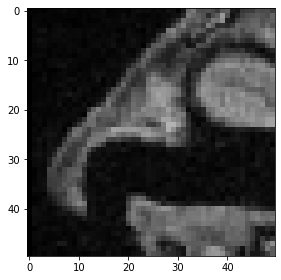And this is how a maximum-intensity projections of this cropped cube look like.

maximum_intensity_projection_along_z = np.max(cropped_cube, axis=0)
imshow(maximum_intensity_projection_along_z)

<matplotlib.image.AxesImage at 0x2b550a03f40>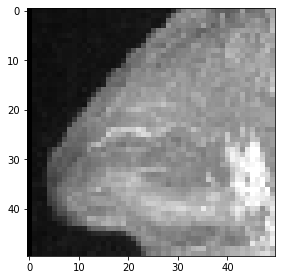maximum_intensity_projection_along_y = np.max(cropped_cube, axis=1)
imshow(maximum_intensity_projection_along_y)

<matplotlib.image.AxesImage at 0x2b550a5e8e0>